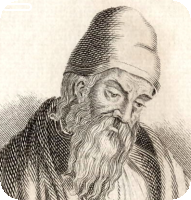# Mathematics

### Prime factors, HCF, LCM and Euclid Algorithm

#### Prime Numbers

Numbers that can only be divided by itself and 1 are called prime numbers.

E.g. 2,3,5,7,9,11,13,.........................

1 is not a prime number; 2 is the only number that is both prime and even.

#### Prime Factors

When the factors of a number is written in the form of prime factors, they are called prime factors.

E.g. 12 - 1,2,3,4,6 and 12 are factors of 12, but they are all not prime factors

However, when 12 is written in terms of 2X2X3, both 2 and 3 are called prime factors.

In order to find prime factors of a number, keep dividing it with prime numbers, starting with the smallest, until you end up with a prime number.

E.g.

240 :-2 = 120 ⇒ 120 :- 2 = 60 ⇒ 60 :- 2 = 30 ⇒ 30 :-2 = 15 ⇒ 15 :- 3 = 5
240 = 2 X 2 X 2 X 2 X 3 X 5
240 = 24 X 3 X 5

Prime factors can be used to find HCF and LCM of two numbers.

#### HCF

1. Find the prime factors of both numbers first.
2. Then take the common prime factors out.
3. Multiply the lowest power of common prime factors; that gives HCF

E.g.1

Find the HCF of 150 and 120.
150 = 2 X 3 X 5 X 5 = 2 X 3 X 52
120 = 2 X 2 X 2 X 3 X 5 = 23 X 3 X 5
The product of lowest power of common factors = 2 X 3 X 5 = 30
So, HCF of 150 and 120 is 30.

E.g.2

Find the HCF of 75 and 120.
75 = 3 X 5 X 5 = 3 X 52
120 = 2 X 2 X 2 X 3 X 5 = 23 X 3 X 5
The product of lowest power of common factors = 3 X 5 = 15
So, HCF of 75 and 120 is 15.

#### LCM

1. Find the prime factors of both numbers first.
2. Then take the highest power of prime factors of both numbers.
3. Multiply the above prime factors together; that gives LCM

E.g.1

Find the LCM of 150 and 120.
150 = 2 X 3 X 5 X 5 = 2 X 3 X 52
120 = 2 X 2 X 2 X 3 X 5 = 23 X 3 X 5
The product of highest power of prime factors = 23 X 3 X 52 = 600
So, LCM of 150 and 120 is 600.

E.g.2

Find the LCM of 150 and 60.
150 = 2 X 3 X 5 X 5 = 2 X 3 X 52
60 = 2 X 2 X 3 X 5 = 22 X 3 X 5
The product of highest power of prime factors = 22 X 3 X 52 = 300
So, LCM of 150 and 60 is 300.

Now, in order to complement what you have just learnt, use the following programme.

 andThis programme uses the world-famous Euclid's Algorithm that was invented around 300 B.C., in order to find the HCF of two numbers. So, I take this opportunity to honour the intellectual giant for providing us with this great mathematical tool.

#### Euclid's Algorithm

Suppose you want to find the HCF of a and b. Here are the steps of the simple algorithm:

1. Input the two numbers - a and b.
2. If a<b exchange a and b.
3. Divide a by b and get the the remainder r.
4. If r is not equal to zero, replace a by b and b by r, then repeat step 3.
5. If r = 0, then b is the HCF of a and b.Maths is challenging; so is finding the right book. K A Stroud, in this book, cleverly managed to make all the major topics crystal clear with plenty of examples; popularity of the book speak for itself - 7th edition in print.

### Recommended - GCSE & iGCSEThis is the best book available for the new GCSE(9-1) specification and iGCSE: there are plenty of worked examples; a really good collection of problems for practising; every single topic is adequately covered; the topics are organized in a logical order.

### Recommended for A LevelThis is the best book that can be recommended for the new A Level - Edexcel board: it covers every single topic in detail;lots of worked examples; ample problems for practising; beautifully and clearly presented.